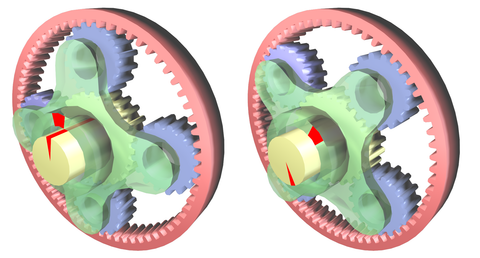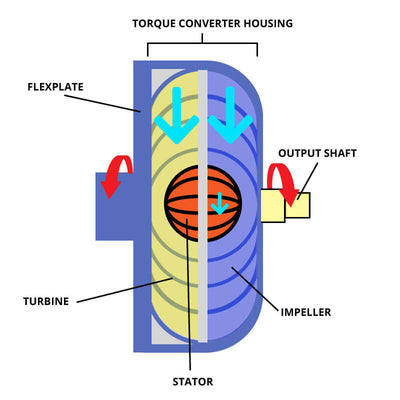Torque Multiplication Factor (TMF) • The All-In-One Guide

Next Gen Drivetrain Research & DevelopmentFebruary 09, 20211 comment

Preface:

Let’s start with the million dollar question. What is a Torque Multiplication Factor (TMF) and why does it matter to me? Before we answer that, it’s important that we understand why this study was performed, and how we accidentally stumbled across this physics goldmine.

Like any company that manufactures any product, we like to ask questions periodically to our clients in the interest of continuously improving our product lineup. We knew that the people most likely to damage the factory transmission were the people most likely to damage an aftermarket one as well, even if less likely. As a result, we performed a study on vehicles that were able damage the factory transmission.

The purpose of the study was simple. We wanted to determine if there was a way to accurately calculate how likely a transmission would be to fail using the same actuarial science that is used to calculate probability in industries like Home or Life Insurance. We based this off of calculable factors such as tire size, differential gear ratio, added mass and more.As a result, we created a proprietary formula to help us understand these data points and apply them accurately, NOT as opinions or simple guesses. This may seem out of place here, but it actually led to an amazing discovery; The vehicles breaking factory transmissions, driveshafts and differentials virtually all had TMF’s above 1:1. In fact, the higher a vehicles TMF, the more likely said vehicle was to damage a drivetrain component, almost proportionately.

Introduction:

So back to the original question — What is the best way to define TMF in reference to automotive drivetrain applications? Well here’s our definition:

Torque Multiplication Factor: A multiplying factor that is used to determine how much added torque is being applied to a vehicles drivetrain; calculated with scientific and mathematical precision by rationalizing factory power against all influential physical forces.

To see it in action, let’s observe an example. Although this technical publication is designed to apply to diesel, gas and EV applications alike, we will use a diesel truck as reference here.

Before beginning the steps necessary to calculate your TMF, you must identify the baseline for your vehicle, the “1:1”. The 1:1 of every vehicle should be the safest and least likely to fail drivetrain configuration available for your truck or car. Overwhelmingly, base model vehicles with no mass-adding features have the best logical projection at life, especially from a drivetrain perspective, as we will further analyze below.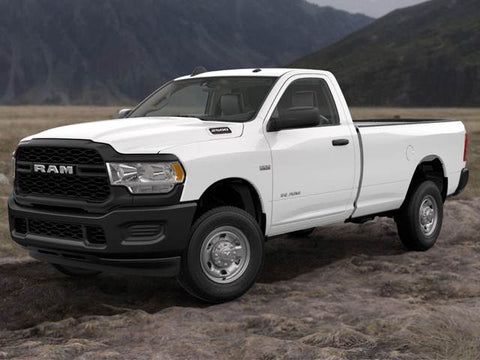In most cases, this is the shortest gear ratio available, the OEM tire size, the unloaded total weight of the vehicle in a standard trim level and factory torque. This is what the vehicle’s power and reliability were originally designed around and hence should be the standard of our analysis.

Now, meet our “Example Truck.” Let’s use a Cummins Turbo-Diesel Equipped 2016 Dodge Ram 3500 with the Aisin Seiki AS69RC transmission. During the purchase process, the customer was offered a choice between 3.42, 3.73 and 4.10 gear ratios inside the differential, opting for the 3.42. 4.10 is the shortest gear ratio here, and 3.42 is the longest.

With very few exceptions, the shortest gear ratio vehicles available tend to have the best longevity, due to their naturally low TMF’s. Also, the OEM tire is a roughly 31.5 inch tire. Lastly, the truck base model 6,400lbs even with the fluids in it. These units of information allow us to establish our “1:1”. This “Example Truck” likely looks much like this:Step #1 - Differential Ratio (Skip for EV’s)

Think about how it’s harder to ride a mountain bike up a hill in a taller gear than it is in a shorter gear. The concept here is that the longer gear ratio necessitates more effort to displace the vehicle the same distance.

This is the concept behind this first step, to account for the resistance created by your differential system. Resistance in mechanical engineering is the force acting to keep an object from moving with other external forces, and translates directly into drivetrain stress.

The first step to calculating TMF accurately is understanding your own gear ratio and tire size (as will be mentioned soon) and how it impacts the survival and behavior of your transmission. To do this is very simple, take the shortest gear ratio available for your year of vehicle and divide it by the one that one has.

Using our example truck, let’s say the gear ratio was a 3.42. This would mean we must divide the gear ratio of 4.10 by 3.42, the equation for this would look roughly as follows:

Formula: A / B = C

4.10 / 3.42 = 1.099

C = 1.099

Key:
A - Shortest OEM Gear Ratio
C - Differential Factor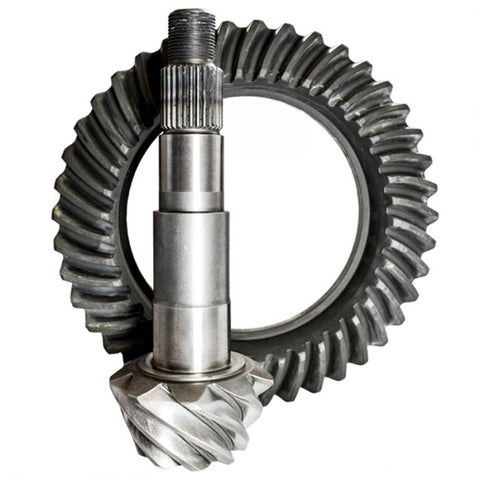Step #2 - Tire Size (Skip if Unmodified)

The second step to accurately calculating TMF is understanding the relationship between the size of your vehicles tires and how it impacts applied torque. This is the same concept as the “Mountain Bike” analogy presented when discussing differential ratio.

Hence, we can use the formula below to calculate the impact of tire size as a metric of resistance as well. In much the same way we calculated the TMF of the differential itself, we will apply a similar formula to tire size. Let’s presume that our example truck uses 37 inch tires.

(A • π) / (B • π) = C

(37 • 3.14159) / (31.5 • 3.14159) = C

116.239 / 98.960 = 1.175

C = 1.175

Key:
A - Your Current Tire Size
B - Your OEM Tire Size
C - Tire Factor
π - Pi (3.14159)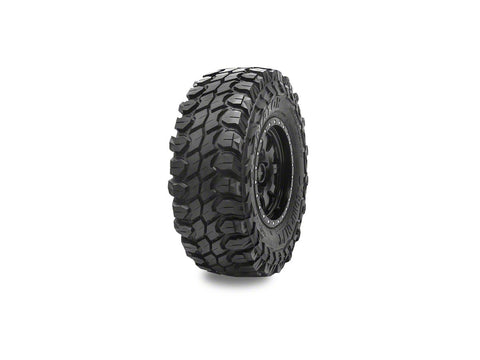Step #3 - Added Mass (Skip if No Notable Weight Added or Subtracted)

The third step in the process is understanding the relationship between both weight reduction and weight addition. In most large trucks, the adjustment here is for the addition of something like a welding assembly or flatbed. Whereas in many gas and electric vehicles, weight reduction is the more common practice.

This is the third and final metric of resistance in most daily driven vehicles. Using the referenced example truck, we will be comparing a Mega Cab Laramie with 4WD to a Tradesman Model with 2WD. To calculate the factor for ones mass rational to factory, the following formula can be applied:

Formula: A / B = C

7800 / 6400 = 1.22

C = 1.22

Key:
A - Total Vehicle Mass as Configured (lbs)
B - Total Vehicle Mass compared to Base Model (lbs)
C - Vehicular Mass FactorStep #4 - Added Motor Power (Skip if Stock)

The final data point necessary in calculating TMF is recognizing the difference in power between your vehicle and the factory’s original design intent. This is how we accounted for added torque. It is only logical that a truck with 2,000ft.lbs. of torque is more apt to damage something than one with 800ft.lbs.

It is important that we be honest with ourselves about numbers here, rather than just basing our figures off of our “economy tune” because we seldom use the upper tunes. If the power is there, it must be accounted for accurately.

Note: If you have not dyno’d your truck to see the exact power figure, use your most accurate and educated guess. Knowing your TMF roughly is far better than not knowing it at all.Using this logic, we created the final part of the formula; The part that accounts for added torque. At the end of the day, torque is what breaks drivetrain components, more traceably than horsepower.

As is common in the diesel community, let’s say this Ram 3500 is equipped with an EZ Lynk tuner yielding an additional 150HP and 300ft.lbs. of torque. To accurately acknowledge the difference in power accurately, use the formula below:

Formula: A / B = C

1100 / 800 = 1.375

C = 1.375

Key:
A - The Amount of Torque you can Produce
B - The Amount of Torque produced from FactoryStep #5 - Crunch the Numbers!

Now for the fun (and heartbreaking) part. Using the Commutative Property of Multiplication, our ability to multiply factors in any order and receive consistent results, we must multiply the TMF of the preceding 4 steps in any order. The formula we use is as follows:

A • B • C • D = E

1.099 • 1.175 • 1.22 • 1.375 = 2.166

TMF (E) = 2.166

Key:
A - Differential Factor
B - Tire Factor
C - Mass Factor
D - Power Factor
E - TMF

Now I have my TMF! But what does it mean?

The final step is simple, take your factory rated torque number and multiply your TMF by that figure. This number will represent the amount of applied torque at the crankshaft. Applied torque is simply how much torque is actually being applied to a specific component after all factors of underdriving and overdriving and otherwise are taken into consideration.In this case, the example truck began life with 800ft.lbs. of torque. Upsizing tires, deleting the truck and simply having a heavier trim selection more than doubled the applied torque of this truck compared to an unmodified base model. Below is the formula for calculating applied torque using your TMF:

A • E = N

800 x 2.166 = 1,733

N = 1,733ft.lbs. of Torque

Key:
A - The Amount of Torque Produced from the Factory
E - TMF
N - Applied Torque at Crankshaft

Conclusion:

By simply rationalizing our easy to calculate TMF to our factory torque rating, we can accurately identify how much torque is actually being applied to our drivetrain components. This is a critical metric for understanding the right transmission parts for our personal application.

It is imperative when researching a transmission or transmission modification to understand at length how this concept works because vehicles (specifically diesel trucks)
with TMF’s in the 1.1 to 1.4 range tend to have substantially shorter lives, with vehicles in the 1.5 and above range being exceedingly more likely to fail within their first 150,000 miles. Vehicles above a factor of 2 are generally only alive due to the conservatism of the driver or the infancy of the power.

It is easier than ever before to find the right transmission or transmission parts for you, and with the least amount of “guesswork” possible. In fact, our  experts would love to educate you on this topic and help you understand your vehicles unique needs.

After reviewing all of this information, it should be very easy to see how simple modifications to your vehicle can yield large impacts in its future and longevity.  If you need help crunching these numbers, we’d love to help. Try giving us a call!